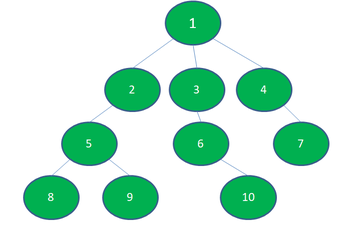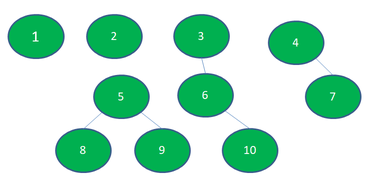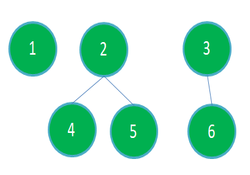Related Articles

# Minimum number of Nodes to be removed such that no subtree has more than K nodes

• Difficulty Level : Medium
• Last Updated : 15 Jun, 2021

Given a tree with N nodes value from 1 to N and (N – 1) Edges and a number K, the task is to remove the minimum number of nodes from the tree such that every subtree will have at most K nodes. Removing Nodes will remove the edges from that nodes to all the other connected nodes.

Examples:

Attention reader! Don’t stop learning now. Get hold of all the important DSA concepts with the DSA Self Paced Course at a student-friendly price and become industry ready.  To complete your preparation from learning a language to DS Algo and many more,  please refer Complete Interview Preparation Course.

In case you wish to attend live classes with experts, please refer DSA Live Classes for Working Professionals and Competitive Programming Live for Students.

Input: N = 10, K = 3, Below is the graph:Output:
Number of nodes removed: 2
Removed Nodes: 2 1
Explanation:
After removing the nodes 1 and 2, here, no subtree or tree has more than 3 nodes. Below is the resulting graph:Input: N = 6, K = 3, Below is the graph:Output:
Number of nodes removed: 1
Removed Nodes: 1
Explanation:
After removing the nodes 1, here, no subtree or tree has more than 3 nodes. Below is the resulting graph:Approach: The idea is to observe that the number of nodes in the subtree of a node X is the sum of the number of nodes in the subtree of its children and the node itself. Below are the steps:

• Use Dynamic Programming and DFS to store the count of nodes in the subtree of each node easily.
• Now, to have no node with subtree having more than K nodes, the idea is to remove the node whenever it has more than K nodes in its subtree, and pass 0 to its parent.
• In the above step, we are having each node with nodes in its subtree not greater than K and minimizing the number of node removals.

Below is the implementation of the above approach:

## C++

 `// C++ program for the above approach``#include ``using` `namespace` `std;` `#define N 20` `// Function to perform DFS Traversal``int` `dfs(vector<``bool``> &visited, ``int` `s,``        ``int` `&K, ``int` `&removals,``        ``vector<``int``> &removed_nodes,``        ``vector> &adj)``{``  ` `  ``// Mark the node as true``  ``visited[s] = ``true``;``  ``int` `nodes = 1;` `  ``// Traverse adjacency list``  ``// of child node``  ``for``(``int` `child : adj[s])``  ``{``    ` `    ``// If already visited then``    ``// omit the node``    ``if` `(visited[child])``      ``continue``;` `    ``// Add number of nodes``    ``// in subtree``    ``nodes += dfs(visited, child, K,``                 ``removals, removed_nodes,``                 ``adj);``  ``}` `  ``if` `(nodes > K)``  ``{``    ` `    ``// Increment the count``    ``removals++;``    ``removed_nodes.push_back(s);``    ``nodes = 0;``  ``}` `  ``// Return the nodes``  ``return` `nodes;``}` `// Function to add edges in graph``void` `addEdge(vector> &adj,``             ``int` `a, ``int` `b)``{``  ``adj[a].push_back(b);``  ``adj[b].push_back(a);``}` `// Function that finds the number``// of nodes to be removed such that``// every subtree has size at most K``void` `findRemovedNodes(vector<``bool``> &visited, ``int` `K,``                      ``int` `&removals,``                      ``vector<``int``> &removed_nodes,``                      ``vector> &adj)``{``  ` `  ``// Function Call to find the``  ``// number of nodes to remove``  ``dfs(visited, 1, K, removals,``      ``removed_nodes, adj);` `  ``// Print Removed Nodes``  ``cout << ``"Number of nodes removed: "``       ``<< removals << endl;` `  ``cout << ``"Removed Nodes: "``;``  ``for``(``int` `node : removed_nodes)``  ``{``    ``cout << node << ``" "``;``  ``}``}` `// Driver Code``int` `main()``{``  ` `  ``// Variables used to store data globally``  ``vector<``bool``> visited(N);``  ``int` `K;``  ``int` `removals = 0;``  ``vector<``int``> removed_nodes;` `  ``// Adjacency list representation of tree``  ``vector> adj(N);` `  ``// Insert of nodes in graph``  ``addEdge(adj, 1, 2);``  ``addEdge(adj, 1, 3);``  ``addEdge(adj, 2, 4);``  ``addEdge(adj, 2, 5);``  ``addEdge(adj, 3, 6);` `  ``// Required subtree size``  ``K = 3;``  ` `  ``// Function Call``  ``findRemovedNodes(visited, K, removals,``                   ``removed_nodes, adj);` `  ``return` `0;``}` `// This code is contributed by sanjeev2552`

## Java

 `// Java program for the above approach``import` `java.util.*;` `class` `GFG {` `    ``// Variables used to store data globally``    ``static` `final` `int` `N = ``20``;``    ``static` `boolean` `visited[] = ``new` `boolean``[N];``    ``static` `int` `K;``    ``static` `int` `removals = ``0``;``    ``static` `ArrayList removed_nodes``        ``= ``new` `ArrayList<>();` `    ``// Adjacency list representation of tree``    ``static` `ArrayList > adj``        ``= ``new` `ArrayList<>();` `    ``// Function to perform DFS Traversal``    ``static` `int` `dfs(``int` `s)``    ``{``        ``// Mark the node as true``        ``visited[s] = ``true``;``        ``int` `nodes = ``1``;` `        ``// Traverse adjacency list``        ``// of child node``        ``for` `(Integer child : adj.get(s)) {` `            ``// If already visited then``            ``// omit the node``            ``if` `(visited[child])``                ``continue``;` `            ``// Add number of nodes``            ``// in subtree``            ``nodes += dfs(child);``        ``}` `        ``if` `(nodes > K) {` `            ``// Increment the count``            ``removals++;``            ``removed_nodes.add(s);``            ``nodes = ``0``;``        ``}` `        ``// Return the nodes``        ``return` `nodes;``    ``}` `    ``// Function to add edges in graph``    ``static` `void` `addEdge(``int` `a, ``int` `b)``    ``{``        ``adj.get(a).add(b);``        ``adj.get(b).add(a);``    ``}` `    ``// Function that finds the number``    ``// of nodes to be removed such that``    ``// every subtree has size at most K``    ``public` `static` `void` `findRemovedNodes(``int` `K)``    ``{``        ``// Function Call to find the``        ``// number of nodes to remove``        ``dfs(``1``);` `        ``// Print Removed Nodes``        ``System.out.println(``"Number of nodes"``                           ``+ ``" removed: "``                           ``+ removals);` `        ``System.out.print(``"Removed Nodes: "``);``        ``for` `(``int` `node : removed_nodes)``            ``System.out.print(node + ``" "``);``    ``}` `    ``// Driver Code``    ``public` `static` `void` `main(String[] args)``    ``{``        ``// Creating list for all nodes``        ``for` `(``int` `i = ``0``; i < N; i++)``            ``adj.add(``new` `ArrayList<>());` `        ``// Insert of nodes in graph``        ``addEdge(``1``, ``2``);``        ``addEdge(``1``, ``3``);``        ``addEdge(``2``, ``4``);``        ``addEdge(``2``, ``5``);``        ``addEdge(``3``, ``6``);` `        ``// Required subtree size``        ``K = ``3``;` `        ``// Function Call``        ``findRemovedNodes(K);``    ``}``}`

## Python3

 `# Python3 program for the above approach` `# Variables used to store data globally``N ``=` `20``visited ``=` `[``False` `for` `i ``in` `range``(N)]``K ``=` `0``removals ``=` `0``removed_nodes ``=` `[]` `# Adjacency list representation of tree``adj ``=` `[[] ``for` `i ``in` `range``(N)]` `# Function to perform DFS Traversal``def` `dfs(s):``    ` `    ``global` `removals``    ` `    ``# Mark the node as true``    ``visited[s] ``=` `True``    ``nodes ``=` `1` `    ``# Traverse adjacency list``    ``# of child node``    ``for` `child ``in` `adj[s]:` `        ``# If already visited then``        ``# omit the node``        ``if` `(visited[child]):``            ``continue` `        ``# Add number of nodes``        ``# in subtree``        ``nodes ``+``=` `dfs(child)` `    ``if` `(nodes > K):` `        ``# Increment the count``        ``removals ``+``=` `1``        ``removed_nodes.append(s)``        ``nodes ``=` `0` `    ``# Return the nodes``    ``return` `nodes` `# Function to add edges in graph``def` `addEdge(a, b):` `    ``adj[a].append(b)``    ``adj[b].append(a)` `# Function that finds the number``# of nodes to be removed such that``# every subtree has size at most K``def` `findRemovedNodes(K):` `    ``# Function Call to find the``    ``# number of nodes to remove``    ``dfs(``1``)` `    ``# Print Removed Nodes``    ``print``(``"Number of nodes removed: "``, removals)` `    ``print``(``"Removed Nodes: "``, end ``=` `' '``)``    ` `    ``for` `node ``in` `removed_nodes:``        ``print``(node, end ``=` `' '``)``    ` `# Driver Code``if` `__name__ ``=``=` `"__main__"``:` `    ``# Insert of nodes in graph``    ``addEdge(``1``, ``2``)``    ``addEdge(``1``, ``3``)``    ``addEdge(``2``, ``4``)``    ``addEdge(``2``, ``5``)``    ``addEdge(``3``, ``6``)` `    ``# Required subtree size``    ``K ``=` `3` `    ``# Function Call``    ``findRemovedNodes(K)` `# This code is contributed by rutvik_56`

## C#

 `// C# program for the above approach``using` `System;``using` `System.Collections.Generic;` `class` `GFG{` `  ``// Variables used to store data globally``  ``static` `readonly` `int` `N = 20;``  ``static` `bool` `[]visited = ``new` `bool``[N];``  ``static` `int` `K;``  ``static` `int` `removals = 0;``  ``static` `List<``int``> removed_nodes``    ``= ``new` `List<``int``>();` `  ``// Adjacency list representation of tree``  ``static` `List > adj``    ``= ``new` `List>();` `  ``// Function to perform DFS Traversal``  ``static` `int` `dfs(``int` `s)``  ``{``    ``// Mark the node as true``    ``visited[s] = ``true``;``    ``int` `nodes = 1;` `    ``// Traverse adjacency list``    ``// of child node``    ``foreach` `(``int` `child ``in` `adj[s])``    ``{` `      ``// If already visited then``      ``// omit the node``      ``if` `(visited[child])``        ``continue``;` `      ``// Add number of nodes``      ``// in subtree``      ``nodes += dfs(child);``    ``}` `    ``if` `(nodes > K)``    ``{` `      ``// Increment the count``      ``removals++;``      ``removed_nodes.Add(s);``      ``nodes = 0;``    ``}` `    ``// Return the nodes``    ``return` `nodes;``  ``}` `  ``// Function to add edges in graph``  ``static` `void` `addEdge(``int` `a, ``int` `b)``  ``{``    ``adj[a].Add(b);``    ``adj[b].Add(a);``  ``}` `  ``// Function that finds the number``  ``// of nodes to be removed such that``  ``// every subtree has size at most K``  ``public` `static` `void` `findRemovedNodes(``int` `K)``  ``{``    ``// Function Call to find the``    ``// number of nodes to remove``    ``dfs(1);` `    ``// Print Removed Nodes``    ``Console.WriteLine(``"Number of nodes"` `+    ``                           ``" removed: "` `+``                               ``removals);` `    ``Console.Write(``"Removed Nodes: "``);``    ``foreach` `(``int` `node ``in` `removed_nodes)``      ``Console.Write(node + ``" "``);``  ``}` `  ``// Driver Code``  ``public` `static` `void` `Main(String[] args)``  ``{``    ``// Creating list for all nodes``    ``for` `(``int` `i = 0; i < N; i++)``      ``adj.Add(``new` `List<``int``>());` `    ``// Insert of nodes in graph``    ``addEdge(1, 2);``    ``addEdge(1, 3);``    ``addEdge(2, 4);``    ``addEdge(2, 5);``    ``addEdge(3, 6);` `    ``// Required subtree size``    ``K = 3;` `    ``// Function Call``    ``findRemovedNodes(K);``  ``}``}` `// This code is contributed by Rohit_ranjan`

## Javascript

 ``
Output
```Number of nodes removed: 1
Removed Nodes: 1```

Time Complexity: O(N)
Auxiliary Space: O(N)

My Personal Notes arrow_drop_up